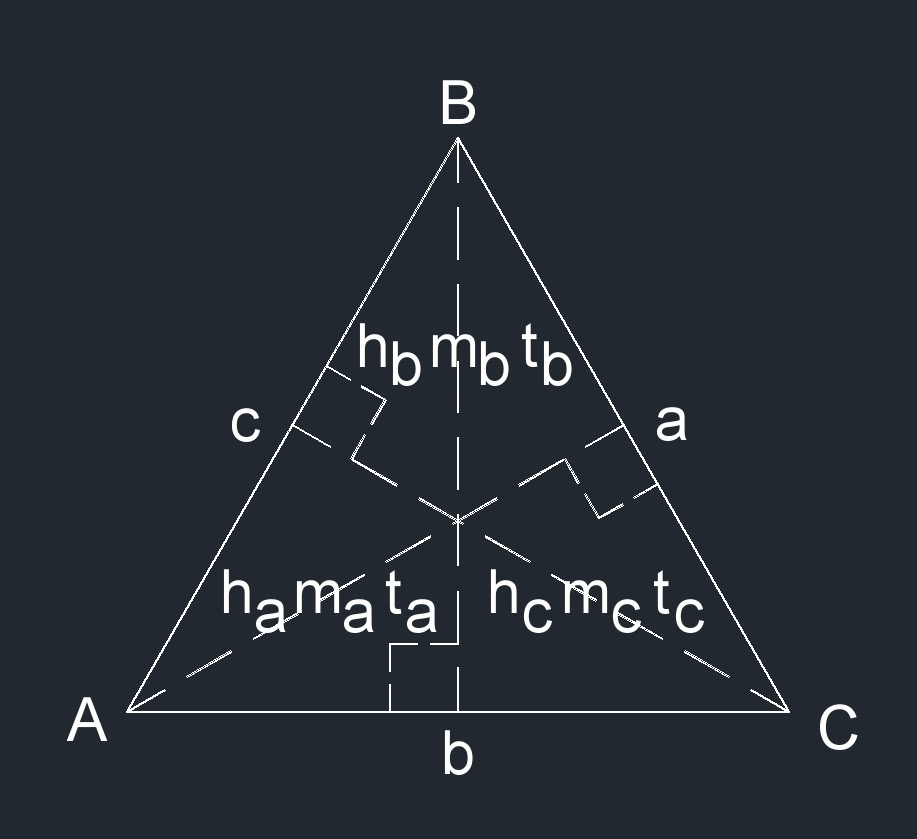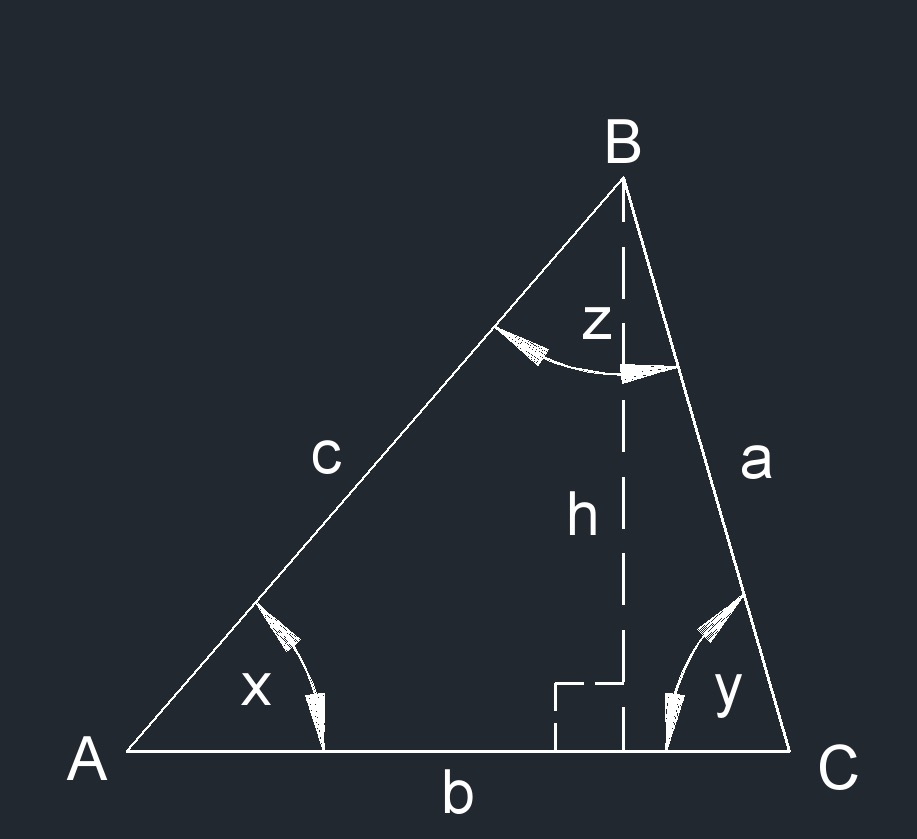# Triangle

Written by Jerry Ratzlaff on . Posted in Plane Geometry

##• All triangles have three angles.
• All triangles have three sides.
• The sum of all angles of a triangle always equal $$180^\circ$$.     $$x^\circ \;+\; y^\circ \;+\; z^\circ = 180^\circ$$
• Sides:  $$a$$,  $$b$$,  $$c$$
• Angles:  $$A$$,  $$B$$,  $$C$$
• Height:  $$h_a$$,  $$h_b$$,  $$h_c$$
• Median:  $$m_a$$,  $$m_b$$,  $$m_c$$  -  A line segment from a vertex (corner point) to the midpoint of the opposite side
• Angle bisectors:  $$t_a$$,  $$t_b$$,  $$t_c$$  -  A line that splits an angle into two equal angles
• Perimeter:  $$P$$
• Semi-perimeter:  $$s$$  -  One half of the perimeter
• Area:  $$K$$
• Inradius of triangle:  $$r$$
• Outradius (circumcircle) of triangle:  $$R$$

## Types of Triangles

### Right Isosceles Triangle• One side of a right isosceles triangle is 90° and two angles are equal.

### Right Triangle• One side of a right triangle is 90°, the other two angles are unequal and no sides are equal.

### Equilateral Triangle• An equilateral triangle is when all three angles are equal.
• A equilateral triangle is a polygon.

### Isosceles Triangle• An isosceles triangle is when two sides are equal and two angles are equal.

### Acute Triangle• An acute triangle is when all three angles of the triangle are less than right angles.
• An acute triangle is a oblique triangle.

### Obtuse Triangle• An obtuse triangle is when one of the three angles is greater than a right angle.
• An obtuse triangle is a oblique triangle.

### Scalene Triangle• An scalene triangle is when no sides and angles are equal.